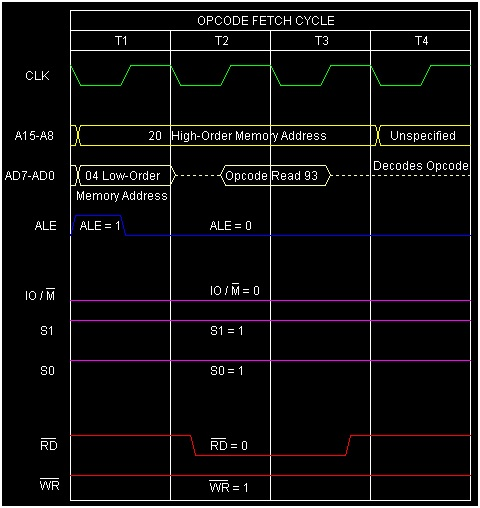# Instruction type SUB R in 8085 Microprocessor

In 8085 Instruction, SUB is a mnemonic that stands for ‘SUBtract contents of R from Accumulator. Here R stands for any of the following registers, or memory location M pointed by HL pair.

R = A, B, C, D, E, H, L, or M


Mnemonics, Operand Opcode(in HEX) Bytes
SUB A 97 1
SUB B 90 1
SUB C 91 1
SUB D 92 1
SUB E 93 1
SUB H 94 1
SUB L 95 1
SUB M 96 1

In this instruction content of the register R will get subtracted from the Accumulator and the resultant difference will be stored on the Accumulator replacing the previous content of the Accumulator. Where R can be any of the eight values, so as a result there are eight opcodes for this type of instruction as mentioned in the above table. It occupies only 1-Byte in the memory.

Let us consider SUB E as an example instruction of this category. As It is 1-Byte instruction so it will occupy a single Byte space in the memory. The result of execution of this instruction is shown below with examples.

## Example 1

Let us supposeAccumulator and E register are having initial values as ABH and CDH respectively. So after execution of the instruction SUB E the contents of the registers would be

Before After

(A)

ABH DEH

(E)

CDH CDH

(F)

Any values Cy=1,AC=0,S=1,P=1,Z=0

2004 93 SUB E Accumulator = Accumulator + 2’s Complement of E

Here Cy=1 indicates that result is negative. Actually A – E = A + 2’ Complement of E. Here is the calculation.

(A)	ABH (1010 1011) ---------------> 1010 1011
2'S Complement
(E)  CDH (1100 1101) --------------->      0011 0011
---------------
1101 1110 (DEH)


Here is the timing diagram of the instruction SUB E as belowSummary − So this instruction SUB E requires 1-Byte, 1 Machine Cycles (Opcode Fetch) and 4 T-States for execution as shown in the timing diagram.

## Example 2

Let us suppose Accumulator and E register are having initial values as CDH and ABH respectively. So after execution of the instruction SUB E the contents of the registers would be

Before After

(A)

CDH 22H

(E)

ABH ABH

(F)

Any values Cy=0,AC=1,S=0,P=1,Z=0

2004 93 SUB E Accumulator = Accumulator + 2’s Complement of E

Here Cy=0 indicates that result is positive. Actually A – E = A + 2’ Complement of E. Here is the calculation.

(A)	CDH (1100 1101) ---------------> 1100 1101
2'S Complement
(E) ABH (1010 1011) --------------->    0101 0101
----------------
0010 0010 (22H)


Here is the timing diagram of the instruction SUB E as belowSummary − So this instruction SUB E requires 1-Byte, 1 Machine Cycles (Opcode Fetch) and 4 T-States for execution as shown in the timing diagram.